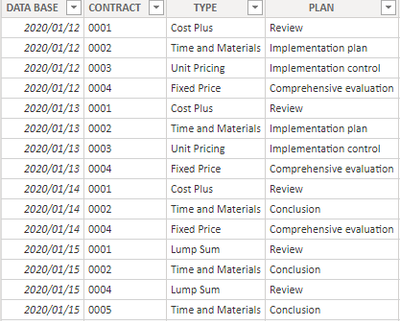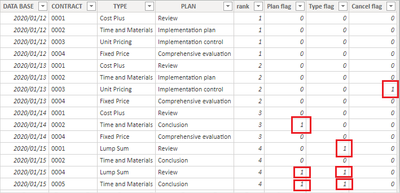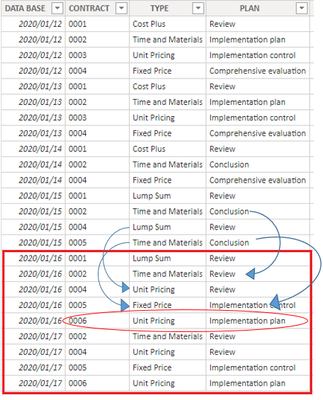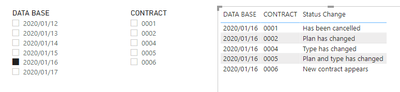cancel
Showing results for
Did you mean:## Dynamic calculation of contract status based on the updated dataset

Scenario:

Suppose I have a table with records of the type and plan of all my contracts based on the date which updated every day.

When the status of my contacts changed, I want to see the detail of the status change in my Power BI reports. (Appeared, Disappeared, Changed). This article explains a method to achieve this using DAX formulas.

Sample Data:

Here's the sample table that contains the data from 2020/1/12 to 2020/1/15Expected output:Guide Line:

To achieve this requirement, we have two main steps:

1. Create the calculated columns [Plan flag], [Type flag] and [Cancel flag] to identify if the plan or type of the contracts have changed.
2. Create a measure [Rank group by CONTRACT] to identify if there are new contracts appeared or old contracts cancelled.
3. Create the measure [Status Change] using the SWITCH() function to give corresponding comment for each type of status change based on the value of the calculated columns and measures I created before.

Operations:

1. Create calculated columns to identify if the plan or type of the contracts have changed.

1) Create a [rank] column to rank the data by Date:

``rank = RANKX('Table','Table'[DATA BASE],,ASC,Dense)``

2) Create the calculated columns [Plan flag] , [Type flag] and [Cancel flag]. If the value didn’t change, they display 0, if the value changed, they display 1.

``````Plan flag =
var _lastPLAN=
CALCULATE(
MAX('Table'[PLAN]),
FILTER('Table',
[CONTRACT]=EARLIER([CONTRACT])&&
[rank]=EARLIER([rank])-1 ))
return
IF(
[rank]<>1,
IF([PLAN]=_lastPLAN,0,1),
0
)``````

``````Type flag =
var _lastTYPE=
CALCULATE(
MAX('Table'[TYPE]),
FILTER('Table',
[CONTRACT]=EARLIER([CONTRACT])&&
[rank]=EARLIER([rank])-1 ))
return
IF(
[rank]<>1,
IF([TYPE]=_lastTYPE,0,1),
0
)``````

``````Cancel flag =
var _maxrank=
MAXX(ALLSELECTED('Table'),[rank])
var _contractsnextday=
SELECTCOLUMNS(
FILTER('Table',
[rank]=EARLIER([rank])+1),
"Contract",[CONTRACT])
return
IF(
[rank]<_maxrank,
IF(
[CONTRACT] in _contractsnextday,
0,1),0
)``````

The output of these calculated columns in the table is like this:As we can see from the table, when the [TYPE] or [PLAN] of these contracts is different from what they are one day before, the flag column will become 1. When the contract disappears in the table, the last row of this contract in the table will become 1. This is the logic I want to implement in these columns.

1. Create a measure [Rank group by CONTRACT] to get the number of times the contract row appears in the table
``````Rank group by CONTRACT =
RANKX(
FILTER(ALL('Table'),
[CONTRACT]=MAX([CONTRACT])),
CALCULATE(MAX('Table'[DATA BASE])),,ASC,Dense
)``````

When I put the measure into a table chart, the output is like this:As we can see, for newly appearing contracts, the value of this measure starts at 1.

1. Create a measure [Status Change] to get the status change of all changed contracts:
``````Status Change =
SWITCH(
TRUE(),
[Rank group by CONTRACT]=1,"New contract appears" ,
MAX('Table'[Type flag])=1&&MAX('Table'[Plan flag])=1,"Plan and type has changed",
MAX('Table'[Type flag])=1,"Type has changed",
MAX('Table'[Plan flag])=1,"Plan has changed",
MAX('Table'[Cancel flag])=1,"Has been cancelled",
BLANK()
)``````

In this DAX formula, I used SWITCH() function to give corresponding comment for each type of status change. If the status stays as it was, I gave it blank value.

Output:

This is the expected table chart that records all the status changes. To make the data clearer and easier to classify, I created two slicers to slice the data based on Date and Contract number:To test the function, I updated my dataset to add the data of 2020/1/16 and 2020/1/17, this is the new data inserted to the table:As we can see, in the data of 2020/1/16, there are some changes on the contract status:
For 0002, Plan changed;

for 0004, Type changed;

for 0005, Plan and Type both changed;

In the data of 2020/1/17, 0001 disappeared.

Then I go to the report, slice the data to“2020/01/16”, the output displayed like this:Author:  Robert Qin

Reviewer: Ula Huang, Kerry Wang

Top Kudoed Posts
Latest Articles
Archives
Polls
What is your favorite Power BI feature release for September 2023?